[] S.Y. Chen and Y.F. Li "Determination of Stripe Edge Blurring for Depth Sensing", IEEE Sensors Journal, Vol. 11, No. 2, Feb. 2011, pp. 389-390.

* This file is only for search engines. Please obtain the formally published article from the respective publisher or databases.

Determination of Stripe Edge Blurring for Depth Sensing

?/span>

 Determination of Stripe Edge Blurring for Depth Sensing

 S. Y. Chen, Senior Member, IEEE, and Y. F. Li, Senior Member, IEEE

Abstract—Estimation of the blurring effect is very important for many imaging systems. This letter reports an idea to efficiently and robustly compute the blurring parameter on certain stripe edges. Two formulas are found to determine the degree of imaging blur only by calculating the area sizes under the corresponding profile curves, without the need for deconvolution or transformation over the image. The method can be applied to many applications such as vision sensing of scene depth. A 3D vision system is taken as an implementation instance.

Index Terms?/span>Point spread function, stripe edge blurring, computer vision, image processing, 3D reconstruction

# I.     INTRODUCTION

 V

ision is one of the most important senses of living beings and about 80% of human information is obtained by vision. In machine, vision is similarly effective for depth sensing or three-dimensional (3D) reconstruction of the environment or target since we are in a 3D space . This letter reports an idea how to formulate an efficient and reliable method for determination of stripe edge blurring from images for vision sensing of scene depth. The method is initially based on analysis of point spread function (PSF) which describes the response of an imaging system to a source point, which often causes blurring of object edges -. The function defines how a point source would appear if imaged with the device. For a camera or projector, the effects are reflected on the image plane of certain distances .

In the literature, the existing contributions have mainly attempted the following methods for image deblurring: (a) image sharpness, (b) peak signal to noise ratio, and (c) blind deconvolution. The first two methods are based on a global operation and often need a reference image. In most cases, we are usually not able to apply a nonblind deconvolution technology . It is always desired to reliably identify the blurring based on PSF estimation.

This letter promises to formulate a function for analysis of stripe edge blurring based on fast PSF computation. It can be used for many purposes in different sensing applications, e.g. (a) to estimate the degree of image blur, for image evaluation and restoration, (b) to find the edge position in sub-pixel precision, for applications of accurate measurement and super-resolution, and (c) to determine the out-of-focus displacement, as a cue of scene depth. Although it is a general method, this letter takes the 3D sensing system as an implementation instance.

# II.     Stripe Edge Blurring and Depth Sensing

## A.     Stripe edge blurring

In this letter, we concern the blurring in a vision sensing system mostly caused by out-of-focus. If with traditional methods, the blur radius s in a specific image point has to be computed by numerical integration and Fourier transform of the image intensity curve along the orthogonal direction of the stripe edge. For example, the function I(x) on a stripe edge is illustrated in Fig.1a. I0 is the theoretical or maximum intensity on the stripe edge. It is, of course, very complicated and inefficient.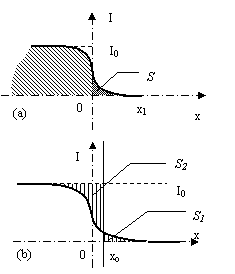Fig. 1?The irradiant flux of blurred area and its computation model. (a) The profile curve caused by out-of-focus blur on a stripe edge, (b) determination of the blur radius.

This letter proposes a formula to determine the blur radius with low computational cost and high precision. With a step light projected on the object surface, the blur radius is proportional to the time rate flow of irradiant light energy in the blurred area, i.e.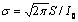, where S is the area size as illustrated in Fig. 1a. This can be proved in the following way.

In the one-dimensional case, consider the illumination whose intensity profile is a step function (Fig. 1a). The brightness on the illuminated scene is the convolution of the PSF and source intensity curve, i.e.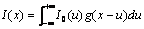=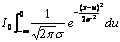?

=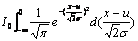=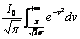.                             (1)

The blurred illumination function has a shape illustrated in Fig. 1a. The area size under this blurring curve is the integration of I(x) from 0 to +?/span>, i.e.?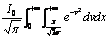=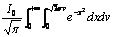=?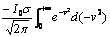?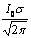. (2)

From (2), we know that the blur radius s is proportional to the area size under the blurring curve: s = 2.5066S/I0.

With this formula, we only need to compute the area size S for every stripe to determine the blur radius. In a simple way, the edge position (where x = 0) is detected firstly by a gradient method, and then S is determined by summating the intensity function from 0 to x1. However, even using a sub-pixel method for the edge detection, errors are still considerable since I(x) changes rapidly around the edge point.

This letter further finds a more accurate method in the sense of energy minimization. As illustrated in Fig. 1b, we have

Fs(xo)=S1(xo)+S2(xo)

=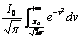+I0-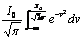.                              ?(3)

It can be proved that the derivative of (3), Fs' ≥ 0, if xo ≥ 0, where "=" holds if and only if xo = 0. The same situation occurs when xo ≤ 0. Therefore, at xo = 0, we have S = min(Fs)/2. This means that the same quantity of light energy flows from S2 to S1. Using this method to compute S is stable and it yields high accuracy. Figure 2 shows an example of the proposed method carried in our experiments. In the system, a pattern of color light stripes is generated by the computer and sent to a digital projector. When the camera captures such an image of illuminated scene, the blur distribution is analyzed according to the formulas proposed in this letter. As the blur radius is proportional to the scene distance, by analysis of every edge points we can calculate the 3D surface by visual sensing of scene depth.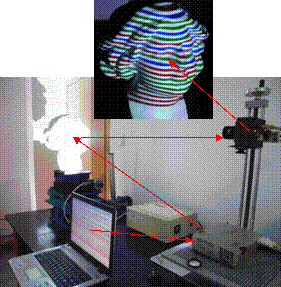Fig. 2?An instance of applications for depth sensing

# III.     Conclusion

This letter proposed a novel method to accurately and efficiently estimate the PSF and blur radius for vision sensing systems. The method mainly includes two formulas, i.e. one for fast determination of stripe edge blurring and the other for finding the accurate solution by an energy minimization function. It does not involve any blind de-convolution or Fourier transform over the whole image. Besides the method can be used to determine the blur degree for image evaluation and restoration, the method is also useful for finding the edge position in sub-pixel precision and it is thus an accurate measurement method in many applications. For instance, since the blur radius can be converted into scene distance, it can be cooperated in a 3D sensing system as shown in this letter.

References

     A. Kolar, A. Pinna, etc., "A Multishutter Time Sensor for Multispectral Imaging in a 3-D Reconstruction Integrated Sensor," IEEE Sensors Journal, vol.9, no.4, pp.478-484, April 2009

     J. Domke, Y. Aloimonos, "Image Transformations and Blurring," IEEE Trans. on Pattern Analysis and Machine Intelligence, vol. 31, pp. 811-823, 2009.

     D. Grois, I. Shcherback, etc., "Theoretical approach to CMOS APS PSF and MTF modeling - evaluation", IEEE Sensors Journal, vol. 6, no. 1, pp.118-124, 2006.

     F. Chen, J. Ma, "An Empirical Identification Method of Gaussian Blur Parameter for Image Deblurring," IEEE Trans. Signal Processing, vol.57, no.7, pp.2467-2478, July 2009.

     S. Y. Chen, Y. F. Li, J. W. Zhang, "Vision Processing for Realtime 3D Data Acquisition Based on Coded Structured Light", IEEE Trans. on Image Processing, vol. 17, pp.167-176, 2008.

     Y. F. Li and S. Y. Chen, "Automatic Recalibration of an Active Structured Light Vision System", IEEE Transactions on Robotics, vol. 19, no.2, pp. 259-268, April 2003.

     J. Ma, F. L. Dimet, "Deblurring From Highly Incomplete Measurements for Remote Sensing," IEEE Trans. on Geoscience and Remote Sensing, vol. 47, 792-802, 2009.

     P. Rusch, R. Harig, "3-D Reconstruction of Gas Clouds by Scanning Imaging IR Spectroscopy and Tomography", IEEE Sensors Journal, Vol.10, No.3, 2010, pp. 599 - 603.

Manuscript received April 25, 2010, revised August 18, 2010. This work was supported by Alexander von Humboldt Foundation, NSFC (60870002), Zhejiang DST (2010R10006, 2009C21008, Y1090592), NCET, RGC-HK (CityU 117507).

S. Y. Chen is with the College of Computer Science, Zhejiang University of Technology, 310023 Hangzhou, China (e-mail: sy@ieee.org).

Y. F. Li is with the Department of Manufacturing Engineering and Engineering Management, City University of Hong Kong, Kowloon, Hong Kong (e-mail: meyfli@cityu.edu.hk).

Digital Object Identifier 2010.20#####